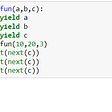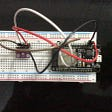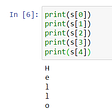# Color Detection using Pandas and OpenCV

`pip install opencv-python numpy pandas`
• Color_detection.py — main source code of our project.
• Color.jpg — sample image for experimenting.
• Colors.csv — a file that contains our dataset.
`import argparseap = argparse.ArgumentParser()ap.add_argument('-i', '--image', required=True, help="Image Path")args = vars(ap.parse_args())img_path = args['image']#Reading image with opencvimg = cv2.imread(img_path)`
`#Reading csv file with pandas and giving names to each columnindex=["color","color_name","hex","R","G","B"]csv = pd.read_csv('colors.csv', names=index, header=None)`
`cv2.namedWindow('image')cv2.setMouseCallback('image',draw_function)`
`def draw_function(event, x,y,flags,param):    if event == cv2.EVENT_LBUTTONDBLCLK:        global b,g,r,xpos,ypos, clicked        clicked = True        xpos = x        ypos = y        b,g,r = img[y,x]        b = int(b)        g = int(g)        r = int(r)`
`def getColorName(R,G,B):    minimum = 10000    for i in range(len(csv)):        d = abs(R- int(csv.loc[i,"R"])) + abs(G- int(csv.loc[i,"G"]))+ abs(B- int(csv.loc[i,"B"]))        if(d<=minimum):            minimum = d            cname = csv.loc[i,"color_name"]    return cname`
`while(1):    cv2.imshow("image",img)    if (clicked):        #cv2.rectangle(image, startpoint, endpoint, color, thickness) -1 thickness fills rectangle entirely        cv2.rectangle(img,(20,20), (750,60), (b,g,r), -1)        #Creating text string to display ( Color name and RGB values )        text = getColorName(r,g,b) + ' R='+ str(r) + ' G='+ str(g) + ' B='+ str(b)        #cv2.putText(img,text,start,font(0-7), fontScale, color, thickness, lineType, (optional bottomLeft bool) )        cv2.putText(img, text,(50,50),2,0.8,(255,255,255),2,cv2.LINE_AA)  #For very light colours we will display text in black colour        if(r+g+b>=600):            cv2.putText(img, text,(50,50),2,0.8,(0,0,0),2,cv2.LINE_AA)        clicked=False    #Break the loop when user hits 'esc' key     if cv2.waitKey(20) & 0xFF ==27:        breakcv2.destroyAllWindows()`

--

--

--

## More from AISHWARIYA SUBAKKAR

Love podcasts or audiobooks? Learn on the go with our new app.

## Dual Path Scripts Interactive and Automated## Easy ETL with Python: a step by step tutorial## Agility & Project Management at Fixed-Cost## A step-by-step guide to finding a code mentor## Try out the try method## Output: Piezo Speakers## voice controlled home automation## How to Create a Writing Portfolio When You Have Zero Experience## What is Generator in Python## External Sensor using ESP32 with BMP280## Introduction To Strings in Python## Battery capacity estimation using Machine Learning : Part-2